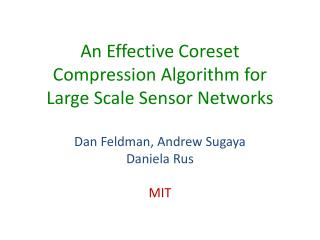DownloadDownload PresentationData

# Data

Télécharger la présentation## Data

- - - - - - - - - - - - - - - - - - - - - - - - - - - E N D - - - - - - - - - - - - - - - - - - - - - - - - - - -
##### Presentation Transcript

1. An Effective Coreset Compression Algorithm for Large Scale Sensor NetworksDan Feldman, Andrew SugayaDaniela RusMIT

2. Data Data Data Data = Data Data Data

3. How much data?

4. 1 GPS Packet = 100 bytes (latitude, longitude, time)

5. 1 GPS Packet = 100 bytes every 10 seconds

6. ~40 Mb / hour or ~1 Gb / day

7. ~1 Gb / day per device

8. ~300 million smart phones sold in 2010 http://mobithinking.com/mobile-marketing-tools/latest-mobile-stats

9. For 100 million devices

10. For 100 million devices ~ 100 petabytes per day

11. ~ 100 thousand terabytes per day

12. 2 terabytes each

13. x50000 / day

14. A lot of data.

15. GPS-points Data • iPhones can collect high-frequency GPS traces • GPS-point = (latitude, longitude, time)

16. Example

17. 3-D Visualization • axes: (latitude, longitude) • axis: time

18. Challenges • Storing data on iPhone is expensive • Transmission data is expensive • Hard to interpret raw data • Dynamic real-time streaming data

19. Key Insight: Identify Critical Points • Approximate the n points by k << n semantically meaningful connected segments

20. Our Approach • Approximate the input GPS-points by connected segments using a k-spline • Output the text description of the endpoints (e.g., using Google Maps)

21. Solution overview • Semantically compress data points • Use coresets • Fit lines to the semantic points • Use splines on coreset • Reverse geo-cite to get directions

22. Definition:-Spline A -spline is a sequence of connected segments in

23. Definition:

24. Definition:

25. Distance to a Set

26. Optimal -spline • over every k-spline

27. Optimal -spline • over every k-spline • is an optimal -splineof if :

28. Problem Statement • Input: set P of n data points in Rd and integer k • Output: optimal k-spline for P that provides semantic compression for large data set P

29. Related Work

30. Our Main Compression Theorem • For every set of points in there is a subset C such that: • The maximum distance between a point in to its closest point in is at most • can be computed in time Example application • The optimal -spline of is an -approximation of • An -approximation for can be computed in time using time algorithm

31. Streaming and Parallel Computation

32. Previous Work for streaming

33. Streaming Compression using merge & reduce p1 p2 p3 p4 p5 p6 p7 p8 p9 p10 p11 p12 p13 p14 p15 p16

34. Our Main Streaming Theorem

35. Parallel computation p1 p2 p3 p4 p5 p6 p7 p8 p9 p10 p11 p12 p13 p14 p15 p16

36. Summary -spline points

37. Compress points: to

38. Compress points: to

39. Compress points: to

40. Compress points: to

41. Compress points: to

42. Compress points: to

43. Compress points: to

44. Compress points: to

45. Compress points: to

46. Compress points: to